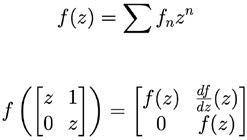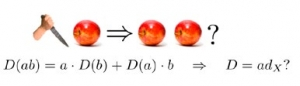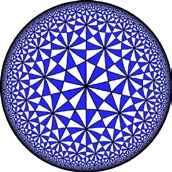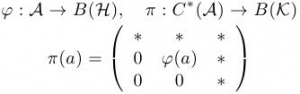## Research faculty members in this area

#### Analytic function spaces and linear operators in several variables

Vector spaces of analytic functions and the linear maps between them are important tools in several branches of Pure and Applied Mathematics. The Hardy Spaces of analytic functions in the complex unit disk, in particular, provide natural models for very general classes of linear operators, and many major themes in Operator Theory, Operator Algebra Theory, and Analytic Function Theory have been inspired by the Hardy Space results.

Motivated by the utility of classical Hardy Space theory, we seek several variable extensions of Hardy Space results and apply them to the modern fields of Multi-variable Operator Theory (the study of linear maps from several copies of a Hilbert Space into itself), Several-variable Complex Function Theory, and Non-commutative Function Theory – a recent and exciting generalization of Complex Analysis and Function Theory to the setting of analytic functions in several non-commuting matrix variables.#### Banach algebras and harmonic analysis

When algebra meets topology, exciting things happen.

Studying a vector space equipped with a norm topology gives rise to the theory of Banach spaces. The topological tool here is tremendously profitable. With it, one is no longer tied with finiteness restrictions. Many Banach spaces that arise naturally have also the ring structure. Studying a ring with an appropriate norm topology leads to the theory of Banach algebras. In a Banach algebra, the algebraic and the topological structures interplay miraculously, defining profound properties of the space. Early research on Banach algebras goes back to the 1930s when J. von Neumann and F. J. Murray launched pioneer investigations on the object. Since then Banach algebras has become a major field in modern functional analysis. The theory developed so far is filled with ingenious ideas and elegant proofs. Standing between analysis and algebra, it has had a deep influence on modern mathematics.

Harmonic analysis is an area dealing with functions and actions of a locally compact topological group. There are many Banach algebras associated with a locally compact group. They reflect intrinsic structure properties of the group. Amenability for a locally compact group is about the existence of a mean (a sort of average operation on bounded functions) that is invariant under translation by the group elements. The theory was established by M. M. Day in 1949 to settle the Banach-Tarski paradox, which states that there is a way to decompose a ball (or to cut an apple) into finite pieces that can be reassembled to get two balls (apples) identical to the original. B. E. Johnson discovered in 1972 that amenability of a locally compact group can be characterized by a class of bounded linear mappings, called derivations, from the group convolution algebra of the group. This initiated the amenability theory for Banach algebras, which has recently been extended further to various generalized notions of amenability for Banach algebras. Amenability of a group/semigroup can also determine common fixed point property of the group/semigroup acting on a subset of a locally convex space. Fixed point theorems have many important applications in many areas of Mathematics.#### Complex analysis and geometric function theory

Complex analysis and geometric function theory studies classes of complex analytic functions. One aspect of geometric function theory is extremal problems, which are connected to some of the deepest themes in analysis: compactness or completeness of families of functions, the Dirichlet principle, variational methods and semigroups. These analytic problems are intimately connected to geometry. For example, one can investigate the geometric properties of extremal maps or the relation between analytic and geometric characterization of classes of analytic maps. Geometric function theory also relates to conformal metrics, hyperbolic geometry, Riemann surfaces and Teichmüller theory.#### Hamiltonian mechanics and integrable systems

Chaos theory traces its origins to Poincaré’s groundbreaking discovery that the equations of motion of 3 massive bodies (e.g. the earth, moon and sun) is non-integrable. Most differential equations that a student may encounter are exactly solvable, or integrable, but these are the exception not the rule. Mathematicians study integrable systems because, like precious gems, their rarity bestows value.

The theory of integrability in dynamical systems is loosely analogous to the theory of solvability of polynomial equations by radicals. The purely algebraic aspect is studied using the tools of differential algebra. However, there are also analytical and even topological aspects to the theory. Today, many fundamental questions remain unresolved. For example, it is not known if being algebraically non-integrable implies analytic non-integrability or the existence of chaos. In many cases, the answer is known, but no general theory exists. It is also not known if chaos can co-exist with analytic integrability, although it is known that chaos and smooth integrability do co-exist.

In addition to their intrinsic worth, integrable systems are useful starting places to understand `near-integrable’ systems. With the tools of KAM theory, for example, it is possible to prove that certain models used in molecular dynamics exhibit paradoxical behaviour: when the temperature is increased, the model predicts the material freezes!

#### Multivariate operator theory and non-self-adjoint operator algebras

This research sits at the interface between complex analysis, functional analysis and operator algebras. The main interest is in the study of bounded linear operators acting on Hilbert space. These are ubiquitous in natural science, appearing as the basic objects of quantum mechanical systems. In the last twenty years or so, there has been a flurry of activity in multivariate operator theory, that is, the study of several operators at a time. At this level of generality, however, not much can be said. Accordingly, concrete operators such as those acting on Hilbert spaces of analytic functions receive considerable attention. Our research centres around the building of bridges between these concrete operators and general abstract ones via the very powerful idea of dilation. This has prompted us to approach operator theory from two distinct angles: either function theoretic through the study of function spaces and their multiplier algebras, or operator algebraic through the consideration of various natural algebras generated by a given operator.#### Operator theory and complex analysis

Operator theory and complex analysis are both parts of analysis and their main goal is exploration of properties of functions defined either on the complex plane or on infinite dimensional spaces of functions. If a function on a vector space is linear, it is also called an operator. The goal is mostly to establish the connections between these two important mathematical objects: functions and operators. While functions have been part of mathematics and other sciences throughout history, the theory of operators is part of modern mathematics that has been historically motivated by its applications to modern physics. Operator theory is also closely related to other more recent, rapidly expanding areas of applied research such as control theory, quantum information and dynamical systems.

Our interests are mostly in two specific classes of operators: Toeplitz and Composition Operators. These operators are related to the basic mathematical operations on functions such as multiplying or composing with a fixed function. The properties of the operator depend on the inducing function and on the space on which they act and are related to the specific requirements for the solutions of a variety of concrete problems in operator theory, complex analysis and mathematical physics.

#### Partial Differential Equations (PDEs)

Our research is in the area of elliptic partial differential equations (PDEs), particularly in semilinear PDEs and in questions related to regularity, existence and multiplicity of their solutions. A lot of the focus has been on the regularity of extremal solutions (stable solutions) and on the related topic of Liouville Theorems for nonlinear PDEs.